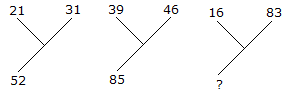# Verbal Reasoning - Character Puzzles - Discussion

### Discussion :: Character Puzzles - Character Puzzles 1 (Q.No.7)

7.

Which one will replace the question mark ?[A]. 92 [B]. 72 [C]. 62 [D]. 99

Explanation:

21 + 31 = 52

and 39 + 46 = 85

Therefore, 16 + 83 = 99.

 Ravikumar said: (Jul 20, 2016) Can anyone tell me one simple logic and tricks to find the solution to these puzzles?